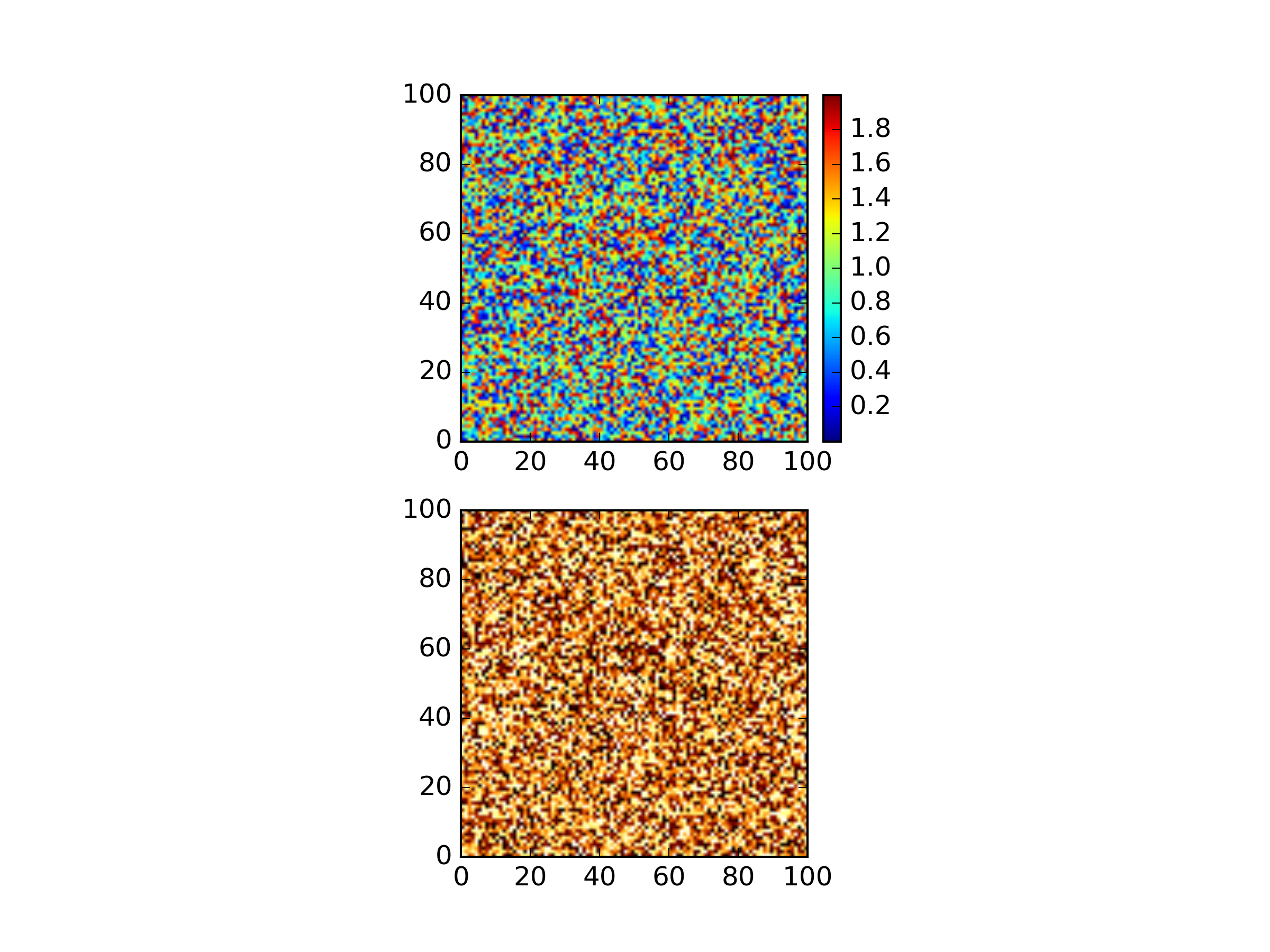# Matplotlib - getting to grips with colorbars and equivalent space

You want to make two plots of two equally sized 2D arrays - one of which has a colorbar, the other not. You also want to ensure that both plots show the arrays at the same equivalent size. This requires the creation of a second axis on each sub-plot

So to walk this through, create data and a new figure:

``````import matplotlib.pyplot as plt
import numpy as np
from mpl_toolkits.axes_grid1 import make_axes_locatable

#make data
extent=[0,100,0,100]

#create figure
fig=plt.figure()
``````

Now create the first subplot and display the distance array:

``````###############
# plot distance

plt.title("Colorbars - to be or not to be")
plt.imshow(distance, extent=extent)
``````

Now create a new axis in which to put the colorbar (which is discussed here):

``````divider = make_axes_locatable(ax)
``````

The colorbar for the current axis is now created:

``````cb = plt.colorbar(cax=cax)
``````

Now create the second subplot and display mask_array:

``````ax2=fig.add_subplot(212) # sets current axis - can use plt calls to modify current axis

``````

We don’t want a colour bar here but we need to create a new axis to ensure the main plot of this sub-plot is in the right place (and doesn’t just fill the whole subplot area):

``````divider = make_axes_locatable(ax2)
``````

As we don’t want a colorbar on this particular subaxis, make stuff on the new axis invisible:

``````cax.set_axis_bgcolor('none')
for axis in ['top','bottom','left','right']:
cax.spines[axis].set_linewidth(0)
cax.set_xticks([])
cax.set_yticks([])
``````

then plot and boom:

``````plt.show()
``````Thanks to Andrew Tedstone for the tip-off!

Written on July 26, 2016